• +91 9971497814
• info@interviewmaterial.com

# Chapter 9 Areas of Parallelograms and Triangles Ex- 9.3 Interview Questions Answers

### Related Subjects

Question 1 :

In Fig.9.23, E is any point on median AD of a ΔABC. Show that ar (ABE)= ar(ACE).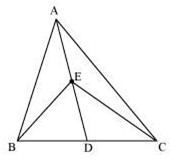Given,

AD is median of ΔABC. , it will divide ΔABC into two triangles ofequal area.

ar(ABD) = ar(ACD) —(i)

also,

ED is the median ofΔABC.

ar(EBD) = ar(ECD) —(ii)

Subtracting (ii) from(i),

ar(ABD) – ar(EBD) =ar(ACD) – ar(ECD)

ar(ABE) = ar(ACE)

Question 2 :

In a triangle ABC, E is the mid-point of median AD. Show that ar(BED)= ¼ ar(ABC).

Answer 2 :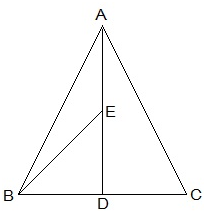ar(BED) = (1/2)×BD×DE

Since, E is themid-point of AD,

AE = DE

Since, AD is themedian on side BC of triangle ABC,

BD = DC,

DE = (1/2) AD — (i)

BD = (1/2)BC — (ii)

From (i) and (ii), weget,

ar(BED) =(1/2)×(1/2)ar(ABC)

ar(BED) = ¼ ar(ABC)

Question 3 :

Show that the diagonals of a parallelogram divide it into fourtriangles of equal area.

Answer 3 :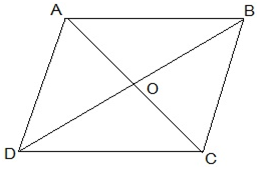O is the mid point ofAC and BD. (diagonals of bisect each other)

In ΔABC, BO is themedian.

ar(AOB) = ar(BOC) —(i)

also,

In ΔBCD, CO is themedian.

ar(BOC) = ar(COD) —(ii)

In ΔACD, OD is themedian.

ar(AOD) = ar(COD) —(iii)

In ΔABD, AO is themedian.

ar(AOD) = ar(AOB) —(iv)

From equations (i),(ii), (iii) and (iv), we get,

ar(BOC) = ar(COD) =ar(AOD) = ar(AOB)

Hence, we get, thediagonals of a parallelogram divide it into four triangles of equal area.

Question 4 :

In Fig. 9.24, ABC and ABD are two triangles on the same base AB. Ifline- segment CD is bisected by AB at O, show that: ar(ABC) = ar(ABD).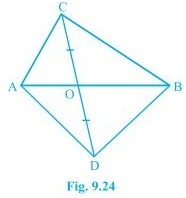In ΔABC, AO is themedian. (CD is bisected by AB at O)

ar(AOC) = ar(AOD) —(i)

also,

ΔBCD, BO is themedian. (CD is bisected by AB at O)

ar(BOC) = ar(BOD) —(ii)

We get,

ar(AOC)+ar(BOC) =ar(AOD)+ar(BOD)

ar(ABC) = ar(ABD)

Question 5 :

D, E and F are respectively the mid-points of the sides BC, CA and ABof a ΔABC.
Show that

(i) BDEF is a parallelogram.

(ii) ar(DEF) = ¼ ar(ABC)

(iii) ar (BDEF) = ½ ar(ABC)

Answer 5 :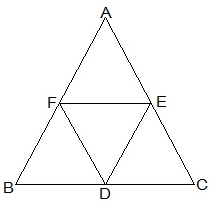(i) In ΔABC,

EF || BC and EF = ½ BC(by mid point theorem)

also,

BD = ½ BC (D is themid point)

So, BD = EF

also,

BF and DE are paralleland equal to each other.

, the pair oppositesides are equal in length and parallel to each other.

BDEF is aparallelogram.

(ii) Proceeding fromthe result of (i),

BDEF, DCEF, AFDE are parallelograms.

Diagonal of aparallelogram divides it into two triangles of equal area.

ar(ΔBFD) = ar(ΔDEF)(For parallelogram BDEF) — (i)

also,

ar(ΔAFE) = ar(ΔDEF)(For parallelogram DCEF) — (ii)

ar(ΔCDE) = ar(ΔDEF)(For parallelogram AFDE) — (iii)

From (i), (ii) and(iii)

ar(ΔBFD) = ar(ΔAFE) =ar(ΔCDE) = ar(ΔDEF)

ar(ΔBFD)+ar(ΔAFE) +ar(ΔCDE) +ar(ΔDEF) = ar(ΔABC)

4 ar(ΔDEF) =ar(ΔABC)

ar(DEF) = ¼ar(ABC)

(iii) Area(parallelogram BDEF) = ar(ΔDEF) +ar(ΔBDE)

ar(parallelogramBDEF) = ar(ΔDEF) +ar(ΔDEF)

ar(parallelogramBDEF) = 2× ar(ΔDEF)

ar(parallelogramBDEF) = 2× ¼ ar(ΔABC)

ar(parallelogramBDEF) = ½ ar(ΔABC)

Question 6 :

In Fig. 9.25, diagonals AC and BD of quadrilateral ABCD intersect at Osuch that OB = OD.
If AB = CD, then show that:

(i) ar (DOC) = ar (AOB)

(ii) ar (DCB) = ar (ACB)

(iii) DA || CB or ABCD is a parallelogram.
[Hint : From D and B, draw perpendiculars to AC.]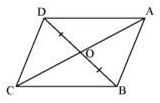Answer 6 :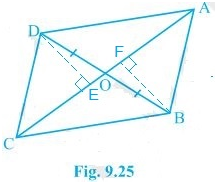Given,

OB = OD and AB = CD

Construction,

DE AC and BF AC are drawn.

Proof:

(i) In ΔDOE and ΔBOF,

DEO = BFO (Perpendiculars)

DOE = BOF (Vertically opposite angles)

OD = OB (Given)

, ΔDOE ΔBOF by AAS congruence condition.

, DE = BF (By CPCT) —(i)

also, ar(ΔDOE) =ar(ΔBOF) (Congruent triangles) — (ii)

Now,

In ΔDEC and ΔBFA,

DEC = BFA (Perpendiculars)

CD = AB (Given)

DE = BF (From i)

, ΔDEC ΔBFA by RHS congruence condition.

, ar(ΔDEC) = ar(ΔBFA)(Congruent triangles) — (iii)

ar(ΔDOE) + ar(ΔDEC) =ar(ΔBOF) + ar(ΔBFA)

ar (DOC) = ar(AOB)

(ii) ar(ΔDOC) =ar(ΔAOB)

Adding ar(ΔOCB) in LHSand RHS, we get,

ar(ΔDOC) + ar(ΔOCB) =ar(ΔAOB) + ar(ΔOCB)

ar(ΔDCB) =ar(ΔACB)

(iii) When twotriangles have same base and equal areas, the triangles will be in between thesame parallel lines

ar(ΔDCB) = ar(ΔACB)

DA || BC — (iv)

For quadrilateral ABCD,one pair of opposite sides are equal (AB = CD) and other pair of opposite sidesare parallel.

, ABCD isparallelogram.

Question 7 :

D and E are points onsides AB and AC respectively of ΔABC such that

ar(DBC) = ar (EBC). Prove that DE || BC.

Answer 7 :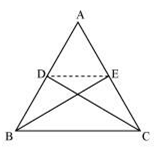Since ΔBCE and ΔBCD arelying on a common base BC and also have equal areas, ΔBCE and ΔBCD will liebetween the same parallel lines.

DE || BC

Question 8 :

XY is a line parallel toside BC of a triangle ABC. If BE || AC and CF || AB meet XY at E and Erespectively, show that

ar(ABE) = ar (ACF)

Answer 8 :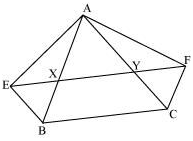It is given that

XY || BC EY || BC

BE || AC BE || CY

Therefore, EBCY is aparallelogram.

It is given that

XY || BC XF || BC

FC || AB FC || XB

Therefore, BCFX is aparallelogram.

Parallelograms EBCY andBCFX are on the same base BC and between the same parallels BC and EF.

Area (EBCY) = Area (BCFX) … (1)

Consider parallelogramEBCY and ΔAEB

These lie on the same baseBE and are between the same parallels BE and AC.

Area (ΔABE) =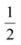Area (EBCY) … (2)

Also, parallelogram BCFX andΔACF are on the same base CF and between the same parallels CF and AB.

Area (ΔACF) =Area (BCFX) … (3)

From equations (1), (2),and (3), we obtain

Area(ΔABE) = Area (ΔACF)

Question 9 :

The side AB of aparallelogram ABCD is produced to any point P. A line through A and parallel toCP meets CB produced at Q and then parallelogram PBQR is completed (see thefollowing figure). Show that

ar (ABCD) = ar (PBQR).

[Hint:Join AC and PQ. Now compare area (ACQ) and area (APQ)]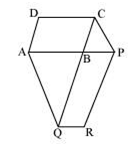Answer 9 :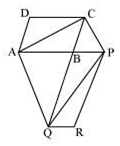Let us join AC and PQ.

ΔACQ and ΔAQP are on thesame base AQ and between the same parallels AQ and CP.

Area (ΔACQ) = Area (ΔAPQ)

Area (ΔACQ) − Area (ΔABQ) = Area (ΔAPQ) − Area (ΔABQ)

Area (ΔABC) = Area (ΔQBP) … (1)

Since AC and PQ arediagonals of parallelograms ABCD and PBQR respectively,

Area (ΔABC) =Area (ABCD) … (2)

Area (ΔQBP) =Area (PBQR) … (3)

From equations (1), (2),and (3), we obtainArea (ABCD) =Area (PBQR)

Area(ABCD) = Area (PBQR)

Question 10 :

DiagonalsAC and BD of a trapezium ABCD with AB || DC intersect each other at O. Provethat ar (AOD) = ar (BOC).

Answer 10 :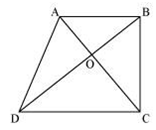It can be observed thatΔDAC and ΔDBC lie on the same base DC and between the same parallels AB and CD.

Area (ΔDAC) = Area (ΔDBC)

Area (ΔDAC) − Area (ΔDOC) = Area (ΔDBC) − Area (ΔDOC)

Area (ΔAOD) = Area (ΔBOC)

Todays Deals### Chapter 9 Areas of Parallelograms and Triangles Ex- 9.3 Contributorskrishan

Name:
Email:

# Latest News# 9000 interview questions in different categories